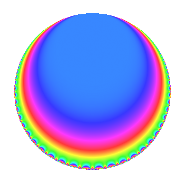Properties

 Label 144.1.gLevel 144 Weight 1 Character orbit g Rep. character $$\chi_{144}(127,\cdot)$$ Character field $$\Q$$ Dimension 1 Newforms 1 Sturm bound 24 Trace bound 0

Related objects

Defining parameters

 Level: $$N$$ = $$144 = 2^{4} \cdot 3^{2}$$ Weight: $$k$$ = $$1$$ Character orbit: $$[\chi]$$ = 144.g (of order $$2$$ and degree $$1$$) Character conductor: $$\operatorname{cond}(\chi)$$ = $$4$$ Character field: $$\Q$$ Newforms: $$1$$ Sturm bound: $$24$$ Trace bound: $$0$$

Dimensions

The following table gives the dimensions of various subspaces of $$M_{1}(144, [\chi])$$.

Total New Old
Modular forms 13 1 12
Cusp forms 1 1 0
Eisenstein series 12 0 12

The following table gives the dimensions of subspaces with specified projective image type.

$$D_n$$ $$A_4$$ $$S_4$$ $$A_5$$
Dimension 1 0 0 0

Trace form

 $$q$$ $$\mathstrut +\mathstrut O(q^{10})$$ $$q$$ $$\mathstrut -\mathstrut 2q^{13}$$ $$\mathstrut -\mathstrut q^{25}$$ $$\mathstrut +\mathstrut 2q^{37}$$ $$\mathstrut +\mathstrut q^{49}$$ $$\mathstrut +\mathstrut 2q^{61}$$ $$\mathstrut -\mathstrut 2q^{73}$$ $$\mathstrut -\mathstrut 2q^{97}$$ $$\mathstrut +\mathstrut O(q^{100})$$

Decomposition of $$S_{1}^{\mathrm{new}}(144, [\chi])$$ into irreducible Hecke orbits

Label Dim. $$A$$ Field Image CM RM Traces $q$-expansion
$$a_2$$ $$a_3$$ $$a_5$$ $$a_7$$
144.1.g.a $$1$$ $$0.072$$ $$\Q$$ $$D_{2}$$ $$\Q(\sqrt{-3})$$, $$\Q(\sqrt{-1})$$ $$\Q(\sqrt{3})$$ $$0$$ $$0$$ $$0$$ $$0$$ $$q-2q^{13}-q^{25}+2q^{37}+q^{49}+2q^{61}+\cdots$$